## Thursday, July 31, 2014

### Sharp EL-501X (today) vs TI-35 Plus (1989)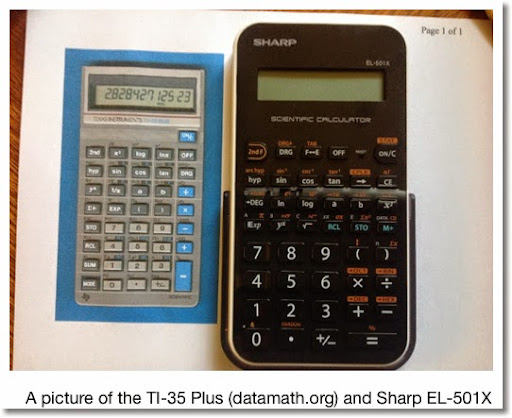TI-35 Plus Notes vs Sharp EL-501X (and almost all of its clones)

Ever wanted a TI-35 Plus? First you could go to eBay or other online stores and try to hunt one down or invest up to \$10 for one of the many close-version that are still manufactured and sold today. There is even a version sold at the Dollar Store! (The keyboard of that \$1 Store I bought is crappy.) Today I will be comparing the Sharp EL-501X against the 1989 TI-35 Plus (and its solar sister, TI-36 Solar (no X)).

Since I never actually owned a TI-35 Plus, all the information will be from its manual. I did buy a TI-36 Solar guidebook, 20 years ago or so, from a clearance bin at Toys R Us of all places. The Sharp EL-501X I bought this week primarily for this blog entry, and to replace a blue cased EL-501X I gave to my cousin's daughter.

Source of the TI-35 Plus information: www.datamath.org

TI-35 Plus Page: http://www.datamath.org/Sci/Modern/TI-35-PLUS.htm

Side plug: Datamath is an excellent web page for all things Texas Instruments calculators, past and preset.

Commonalities

Internal Digits: 12

Modes:
Computational
Complex Mode
Base Display Modes: Binary, Decimal, Octal, Hexadecimal
One Variable Statistics

Complex Mode is limited to arithmetic. The real part is stored and recalled using the [ a ] key and the [ b ] key is for the imaginary part. ( a + bi )

Base Display Modes (except for Decimal) is limited to arithmetic. To convert numbers, just call their respective mode.

Number of memory registers: 1

Factorial Function: n!. Only takes positive integers or zero unless an error occurs.

Polar/Rectangular Conversions:
[ a ] is used for x and r
[ b ] is used for y and θ

Basic Scientific Function set: hyperbolic functions, trigonometric functions and inverses, exponential functions and inverses, reciprocal, square root, cube root, power, arithmetic, π, 3-decimal random number (usually labeled RND)

Differences

Display:
TI-35 Plus: 10 digits plus 2 digit exponent
EL-501X:10 digits or 8 digit plus 2 digit exponent

Exchange Key:
TI-35 Plus has two: EXC to change the number in the display with the number in the memory register. x<>y exchanges operands in power, root, division, and subtraction calculations.
EL-501X: Only the latter is present, often symbolized by a vertical line with up and down arrows. ( [2ndF] [ ( ] )

Decimal Display Settings:
TI-35 Plus: Four permanent modes: floating, fixed, scientific, engineering
EL-501X: Two permanent modes: floating and fixed. Numbers can be toggled between floating/fixed and scientific notation by pressing [ F ←→ E ]

Things the TI-35 Plus had that the EL-501X and most of the clones do not:

Permutations and Combinations. The TI-35 Plus facilitated the use of the [ a ] and [ b ] keys.

Normal Cumulative Distribution Functions (mean = 0, variance = 1, given z):
R(z): area between 0 and z
Q(z): area from z to positive infinity (upper tail)
P(z): area from negative infinity to z (lower tail)

In more advanced calculators and mathematical software, the normalcdf function calculates P(z) (lower tail).

One variate of the EL-501X, the Canon F-604 has permutations, combinations, memory exchange, and fractions. Unfortunately the one I bought not to long ago, the zero key reseted the calculator, so I had to return it. Hopefully it is not the case with all F-604s.

Yes - entry level scientific calculators still exist in the market.

Take care everyone, thanks for comments and questions. Much appreciated. Eddie

This blog is property of Edward Shore. 2014

### Roots of the Cubic Equation - Pythonista 2.7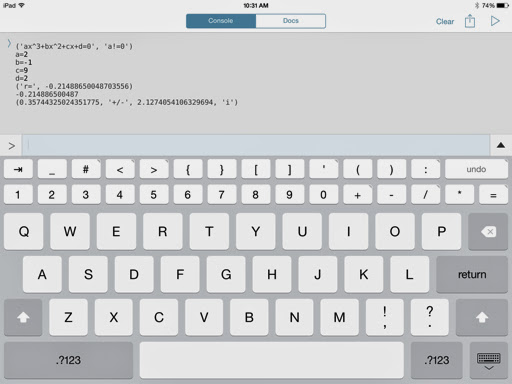Roots of the Cubic Equation - Pythonista 2.7

Script:

# Cubic Equations: 2014-07-30 EWS
# need the root
import math
print('ax^3+bx^2+cx+d=0','a!=0')
a=float(input('a='))
b=float(input('b='))
c=float(input('c='))
d=float(input('d='))
# is a root 1?
test=a+b+c+d
if test==0:
r=1
if test!=0:
# first root by Newton's Method
xn=1
x1=xn-(a*xn**3+b*xn**2+c*xn+d)/(3*a*xn**2+2*b*xn+c)
while math.fabs(xn-x1)>1e-13:
xn=x1
x1=xn-(a*xn**3+b*xn**2+c*xn+d)/(3*a*xn**2+2*b*xn+c)
r=x1
print('r=',r)
# second and third roots
ap=a
bp=a*r+b
cp=a*r**2+b*r+c
disc=bp**2-4*ap*cp
if disc<0:
real=-bp/(2.*ap)
imag=math.sqrt(math.fabs(disc))/(2.*ap)
print(r)
print(real,'+/-',imag,'i')
else:
s=(-bp+math.sqrt(disc))/(2.*a)
t=(-bp-math.sqrt(disc))/(2.*a)
print(r)
print(s)
print(t)

Examples:

x^3 - 4*x^2 - 17*x + 60 = 0
a = 1, b = -4, c = -17, d = 60
Roots: 3, 4, 5

2*x^3 + x^2 - 2*x + 1 = 0
a = 2, b = 1, c = -2, d = 1
Roots (approximately): -1.43756, 0.46878 ± 0.35784i

This blog is property of Edward Shore. 2014

## Saturday, July 26, 2014

### HP Prime: Drawing the Pareto Curve

This program draws the Pareto Curve given a list of frequencies.

Program Name:  PARETO(list of frequencies)

This is best called from the Home Screen and not the program catalog.  The curve drawn is not tracable, but is able to used without having to switch apps.

EXPORT PARETO(flist)
// 2014-07-25 EWS
BEGIN
// frequency list
LOCAL lx,ly,I,J,n;
// Setup
n:=SIZE(flist);
lx:=MAKELIST(X,X,1,n);
ly:=flist/ΣLIST(flist);
ly:=REVERSE(SORT(ly));
ly:=cumSum(ly);
// Draw the Curve
RECT();
Xmin:=0; Xmax:=n+1; Xtick:=1;
Ymin:=0; Ymax:=1.1; Ytick:=0.1;

FOR I FROM 1 TO n-1  DO
LINE(lx(I),ly(I),lx(I+1),ly(I+1),#FFh);
END;

FOR I FROM 1 TO n DO
TEXTOUT(ROUND(ly(I),2),lx(I),ly(I),1,#80h);
TEXTOUT(I,I,0,1,#FF0000h);
LINE(I,0,I,1,#D0D0D0h);
END;

FOR I FROM 0.1 TO 1 STEP 0.1 DO
LINE(0,I,n,I,#D0D0D0h);
END;

WAIT(0);
RETURN ly;
END;

Example:  A list of frequencies:  {4, 8, 9, 5}.  Note that the biggest frequency is plotted first, as the program first arranges the frequencies from largest to smallest.  Each point will show the cumulative percentage of the total population.  A list of the cumulative percentages will be returned to the home screen.Pareto Curve Plot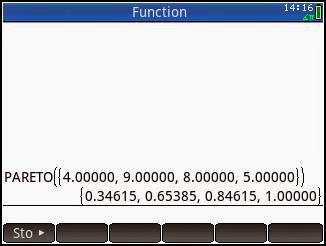List of Cumulative Percentages

Eddie

This blog is property of Edward Shore.  2014

## Friday, July 25, 2014

### Pythonista 2.7: Triangle: Lengths of the side and angle given three points

Pythonista Program: fans.py
Version 2.7

Draws a triangle given three points: S, A, and B with coordinates (sx, sy), (ax, ay), and (bx, by), respectively. Lines formed are SA, SB, and AB. This program finds the lengths of SA, SB, and AB, calculates the angle between SA and SB, and draws the triangle.

Note, the modules math and canvas are used. I think the math module is universal but the canvas module seems to exclusive to Pythonista. Not 100% sure. So if you don't have the canvas module, you can omit the second part of the script out. You will just have text.

Example (see screen shot 2):
S = (10,6)
A = (24,25)
B = (12, -2)

Lengths:
SA = 23.600847442411894
SB = 8.246211251235321
AB = 29.546573405388315
Angle = 129.57940471623766°

Scrpit: fans.py

import math
# find distances and angle
# vertex
print('Vertex Point s')
sx=float(input('sx= '))
sy=float(input('sy= '))
# outer points
print('Point A')
ax=float(input('ax= '))
ay=float(input('ay= '))
print('Point B')
bx=float(input('bx= '))
by=float(input('by= '))
# calculate side lengths sa, sb, and ab
# using the hypot function
sa=math.hypot(sx-ax,sy-ay)
sb=math.hypot(sx-bx,sy-by)
ab=math.hypot(ax-bx,ay-by)
print('|SA|', sa)
print('|SB|', sb)
print('|AB|', ab)
# calcuate the angle between sa and sb
ang=math.acos((sa**2+sb**2-ab**2)/(2.*sa*sb))
ang=math.degrees(ang)
print('Angle beteeen SA & SB:', ang)

# drawing lines - Pythonista
import canvas
# set canvas of 100 x 100 to allow room
canvas.set_size(100,100)
# use pixel (50,50) as the origin
# set stroke line
canvas.set_line_width(.25)
# draw lines
# lines sa and sb are blue
canvas.set_stroke_color(0,0,1)
canvas.draw_line(sx+50,sy+50,ax+50,ay+50)
canvas.draw_line(sx+50,sy+50,bx+50,by+50)
# line ab is dark green
canvas.set_stroke_color(0,0.5,0)
canvas.draw_line(ax+50,ay+50,bx+50,by+50)
# draw axis - gray
canvas.set_stroke_color(0.5,0.5,0.5)
canvas.draw_line(0,50,100,50)
canvas.draw_line(50,0,50,100)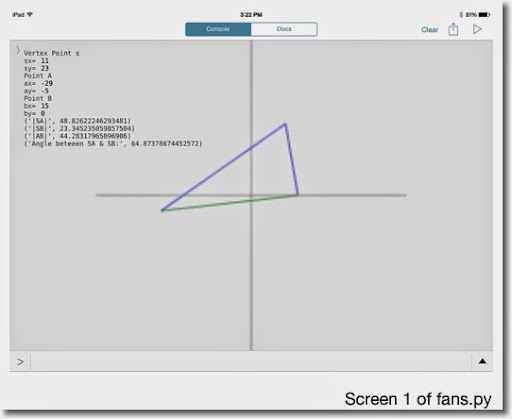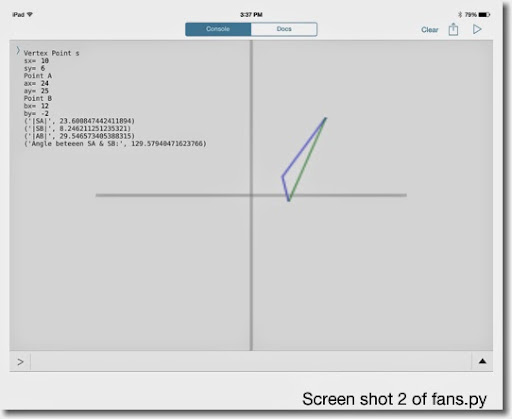Kind of a little intro to Pythonista, at least for me.

Eddie

This blog is property of Edward Shore. 2014

## Tuesday, July 22, 2014

### My experience with Python so far (Pythonista iOS App 2.7)

Overall, my experience with Python has not been a great one, especially trying it with using iOS apps. (Update: but it's getting better - see below)

The iOS Python 3.2 app by Jonathan Hosmer crashes a lot on my iPad (3rd generation). Not worth the \$2.99 in my opinion.

I also tried the pythoni3.3 iOS app by XiaoWen Huang, which is free to download but has in app purchases. Sadly, this app fails in even in giving simple instructions as the help file will tell you everything of the history and how to use it on other machines, but forget it if you want to find how to invoke the interpreter on the app itself. And it crashes if I try to erase the word "in".

Then there is Pythonista 2.7 which has a great interface. However, I am not sure if the mathematics used by this app is not solid or I lack some obvious command. For instance:

Solving 2 by 2 Systems

Solve for x and y:
Ax + By = E
Cx + Dy = F

where:
x = (D*E-B*F)/(A*D-B*C)
y = (A*F-C*E)/(A*D-B*C)

Below is the code for it:
# solving 2 x 2 systems
print('Ax+By=E, Cx+Dy=F')
A=float(input('A='))
B=float(input('B='))
C=float(input('C='))
D=float(input('D='))
E=float(input('E='))
F=float(input('F='))
det=A*D-B*C
x=(D*E-B*F)/det
y=(A*F-C*E)/det
print(det)
print(x)
print(y)

Example:
A = 2
B = 3.5
C = -1
D = 0.4
E = 0.6
F = -2

The results I get are these:
x = 1.68372093023, y = -7.90697674429

Which is fine. I get comparable results when using other calculators and the MathStudio app to verify my answer.

However, the volume of a sphere tells another story.

Volume of the Sphere

Formula = 4/3 * π * r^3

Here is the program and results for r = 3. First the screenshots: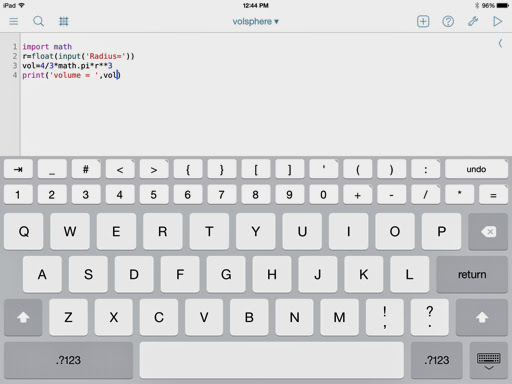Script:

import math
vol=4/3*math.pi*r**3
print('volume = ',vol)

r = 2.45; result Pythonista gives: 46.200654262773026

Verifying the answer with an fx-5800p and HP Prime I get: 61.60087235 (correct answer)

r = 3; result Pythonista gives: 84.82300164692441

However, my calculators return 113.0973355.

Again, am I missing something obvious? Or is Pythonista (maybe the language itself) not reliable?

(Updated) Thank you so much Bhuvanesh Bhatt!

Here is the corrected code:

import math
vol=4/3.*math.pi*r**3
print('volume = ',vol)

In version 2.7, which Pythonista runs, the slash operator (/) is treated as integer divsion. So a decimal point after the denominator is required in order to use floating divsion. In version 3, the extra decimal point is not required.

Under the updated code, I get the correct answers. Thanks once again Bhuvanesh!

Let me know your experiences in the comments below. Thanks as always, Eddie.

This blog is property of Edward Shore. 2014

## Monday, July 21, 2014

### My Mathematical Notes for Python (so far)

Official website: www.python.org

My mathematical notes on Python so far:

This is from the 3.4 instructions but should hold in the 2.7 version as well. I am trying to find a good iOS app to do this.

* A good suggestion is to watch a few tutorial YouTube videos on Python. Onestopprogramming has a good set of tutorials. Python is capable of doing many things, including working with computer files, my focus will be on some of the mathematical capabilities of Python.

* The uses of the equals symbol ( = ) in Python:

(1) A single equal sign means assignment.
x = 4 stores "4" in the variable x.
str = "Eddie" or str = 'Eddie' stores "Eddie" in the variable str.

(2) Two equals signs mean comparison, does x equal y? ( x = y ?)
12==12 returns True

(3) An exclamation mark in front of an equals sign means the comparison, does x not equal y? ( x ≠ y ?)
12!=12 returns False

* Variables that are assigned are defaulted to strings. In order to use variables to represent numbers, we must first declare them as such. The declarations are:

int: integer
long: long integer
float: floating numbers
complex: complex numbers

Example: float(x) declares x as a floating variable.

* Working with floating numbers can bring "weird results" due to Python internally representing every number as a binary representation (0s and 1s). For example,

2.2 * 2 returns 4.40000000000000004

Although the PC version returns 4.4. So this may just be he iOS or a not so good app I was using a the time (Python 3.3 iOS app).

In order to get this answer in more acceptable form. This requires the format declaration. It's syntax is generally this:

Where x is the number of decimal places desired.

Back to our example, let's express 2.2 * 2 using four decimal places:

"{:.4f}".format(2.2*2) returns '4.4000'

* To access mathematical functions beyond arithmetic, we must first import the math library. This is done usually at the beginning of each script (program).

Syntax: import math

Common math functions are what you would expect:

math.ceil(x): ceiling
math.fabs(x): absolute value
math.factorial(x): factorial of x, integers only
math.gamma(x): the gamma function, Γ(x)
math.erf(x): error function
math.fsum(list): sum of a lists elements (in single square brackets)
math.exp(x): e^x
math.log(x, base): logarithm. Leave base out for natural logarithms ( ln x ).
math.pow(x, y): x^y. More expansive than using the double asterisk. ( x ** y )
math.sqrt(x): √x

math.sin(x), math.cos(x), math,tan(x): sine, cosine, and tangent, respectively. The angle is always in radians.
math.asin(x), math.acos(x), math.atan(x): arcsine, arccosine, and arctangent, respectively. The angle returned is in radians.
There are hyperbolic versions of these functions.

math.degrees(x): convert from radians to degrees
math.pi: π
math.e: e

A complete list can be found in the python documentary.

* Quick math symbols that don't need the math library to be imported:

Arithmetic functions: +, -, *, and /. (For those new to programming, * represents × and / represents ÷)

Power: ** (two asterisks)

Modoluo: %

Representing exponential powers of 10: e+N or e-N

That is my quick notes for now. I want to post some programs using the Python language in the upcoming weeks.

Eddie

## Saturday, July 12, 2014

### When is the Glass Half Full?

We have heard the famous two expressions “the glass is half-full” and the “glass is half-empty”; the former is from the view of an optimist while the latter is from the view of a pessimist.  In life, I have been both the optimist and the pessimist.

Let’s consider when a glass is actually half empty, oh sorry, half full.  This is achieved when the volume is half full when the volume of the liquid equals half of the volume of the glass.  Simple enough.

Let q be the flow rate of the liquid being poured in the glass, and assume it is a constant to keep it simple.  (Yes we can a variable flow rate q(t) – this would be appropriate when pouring viscous liquids or considering problems when we are drinking water or our favorite spirit or pop).

With V being the volume of the liquid:

dV/dt = q

dV = q dt

Taking the integral of both sides yield:

V = q*t + V0

Where V0 is the initial volume of the liquid.  Let’s assume that we start with an empty glass.  Then the liquid of the liquid inside of the glass is:

V = q*t

Let C be the volume (or the capacity) of the glass.  The glass is half full when V = C/2 or

t = C/(2 * q)

We can use this equation for various shapes of drinking glasses.  Case in point:For a cylindrical glass:  volume = π * r^2 * h

For a cylindrical glass:  C = π * r^2 * hFor a parabolic glass:  volume = π * r^2 * h/2

For a parabolic glass:  C = π * r^2 * h/2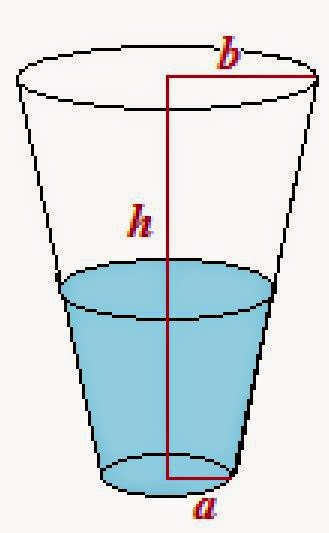For a cup:  volume = π * h/3 * (a^2 + a*b + b^2)

For a cup:  C = π * h/3 * (a^2 + a*b + b^2)

(r, a, and b are radii – half of the distance of the diameter.)

Take the example that we have a cup with dimensions a = 1.375 in, b = 1.75 in, and h = 3.5 in.  We are going to pour iced tea in it at a flow rate of q = 8.3 in^3/sec.

C = π * 3.5/3 * (1.375^2 + 1.375 * 1.75 + 1.75^2) ≈ 26.93752 in^3

and t ≈ 26.97352/(2 * 8.3) ≈ 1.62491 sec

P.S. The flow rate I gave came from a short experiment of actually pouring Arnold Palmer Ice Tea into the glass and timing how long it takes to pour the ice tea, which it took about 3.25 seconds to fill it.  Doing the same experiment with pouring water from a bottle, which took me 7 seconds (a flow rate of approximately 3.9 in^3/sec) – but I think held the bottle closer to the cup.

Pessimists and optimists (and everyone in between) – go out and have an awesome weekend.  Cheers!

Eddie

This blog is property of Edward Shore.  2014.

### HP 15C: Error Function and Lower Tail Normal Cumulative Function

HP 15C:   Error Function and Lower Tail Normal Cumulative Function Formulas Used  Error Function  erf(x) = 2 ÷ √π * ∫( e^(-t^2) dt, t = 0 t...